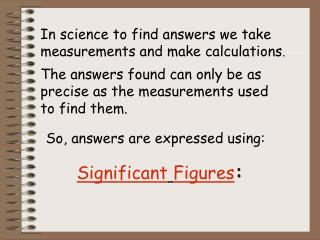DownloadDownload PresentationSignificant Figures :

# Significant Figures :

Télécharger la présentation## Significant Figures :

- - - - - - - - - - - - - - - - - - - - - - - - - - - E N D - - - - - - - - - - - - - - - - - - - - - - - - - - -
##### Presentation Transcript

1. SignificantFigures: In science to find answers we take measurements and make calculations. The answers found can only be as precise as the measurements used to find them. So, answers are expressed using:

2. Significant Figures: Are digits in measurements that are known with certainty plus one estimated digit Ensures that a calculated answer shows only as much precision as the least precise measurement Rely on the measurements taken, to determine how precise your outcome can be.

3. Rule #1: In measurements that do not contain a zero, all numbers are significant. 5.14 3 sig fig Rule #2: All zeros between significant digits are significant. 7.502 4 sig fig Rule #3:.Zeroes to the left of the nonzero digit serve only as place holders and are not significant .056 2 sig fig Rule #4: In a number with digits to the right of the decimal point, zeros to the right of the last non-zero number are significant. 65.400 5 sig fig Rule #5:If the number is a count then sig. fig. is infinite. Measurements without decimal points are ambiguous and more information is needed to determine significant figures. 56 monkeys

4. Determining significant digits in measurements: 3.96 cm 5.0 cg 3.001 m 14pencils . 04 g 7.230 m 219.6 L 45.20 L

5. Significant Figures and Calculated Quantities: Multiplication and Division: the answer must have the number of significant figures found in the measurement with the fewest significant figures. Example:

6. Addition and Subtraction:the answer should not have digits beyond the last digit position common to all numbers being subtracted or added 34.67 40.08 495.87 4.5 40.006 9.5 1.567 40.0005 486.37 120.0865

7. 3.414 sec + 10.02 sec + 58.325 sec + .00098 sec = 2.326 hr – 0.10408 hr = 10.19 m x 0.013 m = 80.23 m / 2.4 sec = 1884. kg + 0.94 kg + 1.0 kg + 9.778 kg = 2104.1 m – 463.09 m = 140.01 cm x 26.042 cm x 0.0159 cm = 4.301 kg / 1.9 cm3 = Practice: